Tamil Nadu Board of Secondary EducationSSLC (English Medium) Class 6th

# Tamil Nadu Board Samacheer Kalvi solutions for Class 6th Mathematics Term 1 Answers Guide chapter 2 - Introduction to Algebra [Latest edition]

#### Chapters## Chapter 2: Introduction to Algebra

Exercise 2.1Exercise 2.2
Exercise 2.1 [Pages 40 - 41]

### Tamil Nadu Board Samacheer Kalvi solutions for Class 6th Mathematics Term 1 Answers Guide Chapter 2 Introduction to AlgebraExercise 2.1 [Pages 40 - 41]

#### Fill in the blanks

Exercise 2.1 | Q 1. (i) | Page 40

The letters a, b, c, … x, y, z are used to represent __________

Exercise 2.1 | Q 1. (ii) | Page 40

The algebraic statement of ‘f decreased by 5’ is ____________

Exercise 2.1 | Q 1. (iii) | Page 40

The algebraic statement of ‘s divided by 5’ is ____________

Exercise 2.1 | Q 1. (iv) | Page 40

If A’s age is ‘n’ years now, 7 years ago A’s age was _____________

Exercise 2.1 | Q 1. (v) | Page 40

If ‘p − 5’ gives 12 then ‘p’ is _____________

#### Say True or False.

Exercise 2.1 | Q 2. (i) | Page 40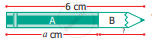The length of part B in the pencil shown is ‘a − 6’

• True

• False

Exercise 2.1 | Q 2. (ii) | Page 40

If the cost of an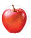is ‘x’ and the cost of a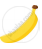is ₹ 5, then the total cost of fruits is ₹ 'x + 5'

• True

• False

Exercise 2.1 | Q 2. (iii) | Page 40

10 more to three times 'c' is '10c + 3'

• True

• False

Exercise 2.1 | Q 2. (iv) | Page 40

If the cost of 10 rice bags is ₹ ‘t’, then the cost of 1 rice bag is ₹ ("‘t'")/10

• True

• False

Exercise 2.1 | Q 2. (v) | Page 40

The product of ‘q’ and 20 is '20q'

• True

• False

Exercise 2.1 | Q 3 | Page 40

Draw the next two patterns and complete the table.Shapes 1st Pattern 2nd Pattern 3rd Pattern 4th Pattern 5th Pattern Squares 1 2 3 Circles 1 2 3 Triangles 2 4 6
Exercise 2.1 | Q 4 | Page 40

Arivazhagan is 30 years younger to his father. Write Arivazhagan’s age in terms of his father’s age

Exercise 2.1 | Q 5. (i) | Page 40

If ‘u’ is an even number, how would you represent the next even number?

Exercise 2.1 | Q 5. (ii) | Page 40

If ‘u’ is an even number, how would you represent the previous even number?

Exercise 2.1 | Q 6. (i) | Page 41

Express the following verbal statement to algebraic statement

Exercise 2.1 | Q 6. (ii) | Page 41

Express the following verbal statement to algebraic statement

4 times ‘q’

Exercise 2.1 | Q 6. (iii) | Page 41

Express the following verbal statement to algebraic statement

4 less to 9 times of ‘y’

Exercise 2.1 | Q 7. (i) | Page 41

Express the following algebraic statement to verbal statement

x ÷ 3

Exercise 2.1 | Q 7. (ii) | Page 41

Express the following algebraic statement to verbal statement

11 + 10x

Exercise 2.1 | Q 7. (iii) | Page 41

Express the following algebraic statement to verbal statement

70s

Exercise 2.1 | Q 8 | Page 41

The teacher asked two students to write the algebraic statement for the verbal statement “8 more than a number”. Vetri wrote ‘8 + x’ but Maran wrote ‘8x’. Who gave the correct answer?

Exercise 2.1 | Q 9. (i) | Page 41

If ‘g’ is equal to 300 what is the value of ‘g – 1’ and ‘g + 1’?

Exercise 2.1 | Q 9. (ii) | Page 41

What is the value of ‘s’, if ‘2s – 6’ gives 30?

Exercise 2.1 | Q 10 | Page 41

Complete the table and find the value of ‘k’ for which ("‘k'")/3 gives 5.

 K 3 6 9 12 15 18 "k"/3 1 2

#### Objective Type Questions

Exercise 2.1 | Q 11 | Page 41

Variable means that it

• can take only a few values

• has a fixed value

• can take different values

• can take only 8 values

Exercise 2.1 | Q 12 | Page 41

The number of days in ‘w’ weeks is

• 30 + w

• 30w

• 7 + w

• 7w

Exercise 2.1 | Q 13 | Page 41

The value of ‘x’ in the circle is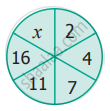• 6

• 8

• 21

• 22

Exercise 2.1 | Q 14 | Page 41

The value of ‘y’ in y + 7 = 13 is

• y = 5

• y = 6

• y = 7

• y = 8

Exercise 2.1 | Q 15 | Page 41

6 less to ‘n’ gives 8 is represented as

• n – 6 = 8

• 6 – n = 8

• 8 – n = 6

• n – 8 = 6

Exercise 2.2 [Pages 42 - 44]

### Tamil Nadu Board Samacheer Kalvi solutions for Class 6th Mathematics Term 1 Answers Guide Chapter 2 Introduction to AlgebraExercise 2.2 [Pages 42 - 44]

#### Miscellaneous Practice Problems

Exercise 2.2 | Q 1 | Page 42

Complete the following pattern.
9 – 1 =
98 – 21 =
987 – 321 =
9876 – 4321 =
98765 – 54321 =
What comes next?

Exercise 2.2 | Q 2. (i) | Page 42

A piece of wire is ‘12s’ cm long. What will be the length of the side, if it is formed as an equilateral triangle

Exercise 2.2 | Q 2. (ii) | Page 42

A piece of wire is ‘12s’ cm long. What will be the length of the side, if it is formed as a square

Exercise 2.2 | Q 3 | Page 43

Identify the value of the shapes and figure in the table given below and verify their addition horizontally and vertically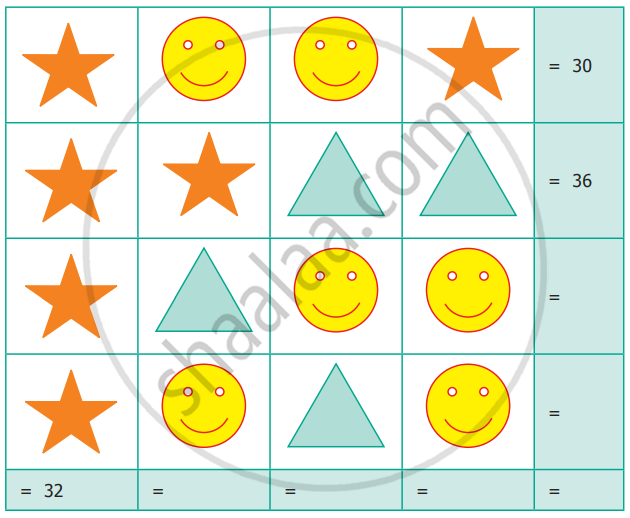Exercise 2.2 | Q 4 | Page 43

The table given below shows the results of the matches played by 8 teams in a Kabaddi championship tournament.

 Teams A B C D E F G H Total Matches played 8 7 n a 9 10 8 y Matches won 5 6 4 7 b 6 x 3 Matches lost k m 6 2 3 c 4 6

Find the value of all the variables in the table given above

#### Challenging Problems

Exercise 2.2 | Q 5 | Page 43

Gopal is 8 years younger to Karnan. If the sum of their ages is 30, how old is Karnan?

Exercise 2.2 | Q 6 | Page 43

The rectangles made of identical square blocks with varying lengths but having only two square blocks as width are given below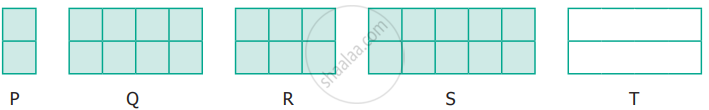(i) How many small size squares are there in each of the rectangles P, Q, R and S?
(ii) Fill in the boxes

 Rectangle P Q R S T Number of small size squares along the breadth 2 2 ? 2 2 Number of squares along the length 1 4 3 ? x Total number of squares in rectangle ? 8 ? 10 ?
Exercise 2.2 | Q 7 | Page 44

Find the variables from the clues given below and solve the cross-word puzzle.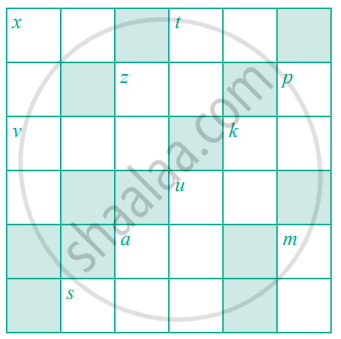Across Down x + 40 gives 100 x is 1005 multiplied by 6 7 reduced from t gives 31 t ÷ 7 = 5 z is 5 added 5 times p is the predecessor of first 3 digit number v is the whole number zero plus number of days in a ordinary year z is the number of weeks in a year (digits reversed) k is 24 added to 25 k is 11 times 4 u is 2 added to two times 11 gives the number of hours in a day u is product of 23 and 9 a is 20 more to 40 a is 4 added to the product of 12 and 5 s minus 1 gives 246 is the number of letters in Tamil language m is the successor of 9

## Chapter 2: Introduction to Algebra

Exercise 2.1Exercise 2.2## Tamil Nadu Board Samacheer Kalvi solutions for Class 6th Mathematics Term 1 Answers Guide chapter 2 - Introduction to Algebra

Tamil Nadu Board Samacheer Kalvi solutions for Class 6th Mathematics Term 1 Answers Guide chapter 2 (Introduction to Algebra) include all questions with solution and detail explanation. This will clear students doubts about any question and improve application skills while preparing for board exams. The detailed, step-by-step solutions will help you understand the concepts better and clear your confusions, if any. Shaalaa.com has the Tamil Nadu Board of Secondary Education Class 6th Mathematics Term 1 Answers Guide solutions in a manner that help students grasp basic concepts better and faster.

Further, we at Shaalaa.com provide such solutions so that students can prepare for written exams. Tamil Nadu Board Samacheer Kalvi textbook solutions can be a core help for self-study and acts as a perfect self-help guidance for students.

Concepts covered in Class 6th Mathematics Term 1 Answers Guide chapter 2 Introduction to Algebra are The Solution of an Equation, Introduction to Algebra, The Idea of a Variable, Expressions with Variables.

Using Tamil Nadu Board Samacheer Kalvi Class 6th solutions Introduction to Algebra exercise by students are an easy way to prepare for the exams, as they involve solutions arranged chapter-wise also page wise. The questions involved in Tamil Nadu Board Samacheer Kalvi Solutions are important questions that can be asked in the final exam. Maximum students of Tamil Nadu Board of Secondary Education Class 6th prefer Tamil Nadu Board Samacheer Kalvi Textbook Solutions to score more in exam.

Get the free view of chapter 2 Introduction to Algebra Class 6th extra questions for Class 6th Mathematics Term 1 Answers Guide and can use Shaalaa.com to keep it handy for your exam preparation